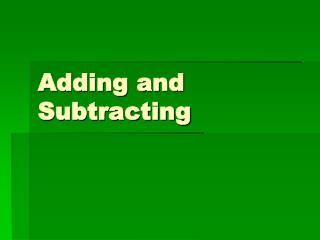DownloadDownload PresentationTélécharger la présentation- - - - - - - - - - - - - - - - - - - - - - - - - - - E N D - - - - - - - - - - - - - - - - - - - - - - - - - - -
##### Presentation Transcript

2. Partial-Sums Method: • Algorithm: is used to name _________________________ procedures for __________________a mathematical problem. *Addition is performed form left to right, column by column *The sum for each column is recorded on a separate line. *The partial sums are added either at each step or at the end. 348 + 177 = ?                               3      4      8  +   1      7      7 400 + 110 + 15 525

3. Partial-Sums Method: Algorithm: is used to name _________________________ procedures for __________________a mathematical problem. *Addition is performed form left to right, column by column *The sum for each column is recorded on a separate line. *The partial sums are added either at each step or at the end.  4.65 + 3.25 = ?                             4.       6     5  +3.       2      5 7 + 0.8+ 0.1 7.9

4. The Column-Addition Method *Each column of numbers is added separately, and in any order. *If adding results in a single digit in each column, the sum has been found. *If the sum in any column is a 2-digit number, it is renamed and part of it is added to the sum in the column on its left. ***This adjustment serves the same purpose as "Carrying" in the traditional algorithm. 359 + 298 = ?                               3      5       9 +2       9       8 Explain the difference once a decimal is added into the problem. What do you have to be sure of?

5. The Trade-First Method • *Problems are written in vertical form. • *If each digit of the minuend  (the larger number) is greater than or equal to the digit directly below it, the problem is very easy to solve.  • *If any digit of the minuend is less than the digit directly below it, then the minuend is adjusted before any subtraction is done. • *The minuend is adjusted by "trading." • 463 - 275 = ?                                      4      6       5 • -2       7        5 • 32.9 - 15.6 = ?                                  3     2.      9 • -1     5.      6 • 16 5 • 2 7 5 • 12. 9 • 1 5. 6

6. The Partial-Difference Method *The subtraction is performed from left to right. *The smaller number in each column is always subtracted from the larger number. *If the bottom number is less than the top number, then the result will be added to obtain the final answer. *If the bottom number is greater than the top number, then the result will be subtracted to obtain the final answer. 4261- 2637 = ?           4 2 6 1 -2 6 3 7 4000 – 2000 = 2000 200 – 600 = (-400) -400 1600 60 – 30 = +30 1630 1-7 = (-6) -6 1624

7. The Partial-Difference Method Let’s try Partial-Sums with Decimals 7 6. 3 8 -3 9. 8 1 What do you think will be the difficulties with decimals using this strategy? What are the benefits? 70 – 30 = 40.00 6 – 9 = (-3) -3 37.00 0.3 – 0.8 = (-0.5) -0.5 36.50 0.08 – 0.01 = 0.07 +0.07 36.57

8. Adding/Subtracting and Place Value When we subtract, we take values away from the larger quantity. Sometimes we need to borrow a ten from the larger place value to cover what is being taken away. 324 -56 I cannot take 6 away from 4, but I can take 6 away from 14. I will borrow a ten from the tens column and add it to the ones column. This does not change the value of the number, but it changes the name of the quantities in the column.

9. Adding/Subtracting and Place Value 324 -56 3 hundreds 2 tens 4 ones = 314 3 hundreds 1 ten 14 ones =314 324 31 14 -56 -5 6

10. Adding/Subtracting and Place Value 324 -56 What if we looked at the problem another way. Can you take 6 away from 24? 24 – 6 = 18 Because of your subtraction, 324 now becomes 318. The new problem is 318 – 50.

11. Adding/Subtracting and Place Value 318-50 Think about what makes sense here. What can you subtract 50 from easily? 300-50=250 Now just combine the differences of the two easier problems you completed. 250 + 18 = 268

12. What About Decimals? How do these strategies apply to decimals? What are the possible problems you will have with these methods?

13. Time to Practice Get with a partner you work well with. Partners will coach each other through these strategies. It is important that you understand how to use each of these strategies before you decide if they work for you or not. Complete both sides of the page. Check in with the teacher when you are finished.